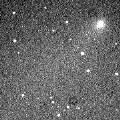# C/1999 L3 ( LINEAR )###Profile

 Discovery Date June 9, 1999 Magnitude 18.3 mag Discoverer Lincoln Laboratory Near-Earth Asteroid Research project

###Pictures###Orbital Elements

```   The following improved orbital elements by Kenji Muraoka, are
from 105 observations 1999 June  9 to 2000 Jan. 18, perturbations
by 9 Planets, Moon and 5 minor planets were taken into account.
The mean residual is +/- 0.54 arc seconds.

Epoch  =  2000 Jan  = 2451560.5
T  =  2000 Jan.  4.90822       +/- 0.00033 (m.e.) TT
Peri. =  353.29761                +/- 0.00039
Node  =  140.16085                +/- 0.00045   (2000.0)
Incl. =  166.09933                +/- 0.00008
q  =    1.9889094              +/- 0.0000059 AU
e  =    0.9743066              +/- 0.0000181
1/a  =   +0.0129184              +/- 0.0000091 1/AU
( P  =    681 years )
```

###Finding Charts###Magnitudes Graph

```        m1 =   6.0 + 5 log d +  19.0 log r  [  , 0]  (             - 2000 Jan.  4)
m1 =  61   + 5 log d - 165   log r  [ 0,30]  (2000 Jan.  4 - 2000 Feb.  3)
m1 = -27   + 5 log d + 125   log r  [30,55]  (2000 Feb.  3 - 2000 Feb. 28)
m1 =   5.5 + 5 log d +  25   log r  [55,  ]  (2000 Feb. 28 -             )

* Gray curve is:  m1 = 6.0 + 5 log d + 19.0 log r
```##### The orbital elements are calculated by Kenji Muraoka. The charts are made with StellaNavigator Ver.2.0 for Windows (AstroArts / ASCII). The magnitudes graphs are made with Comet for Windows.